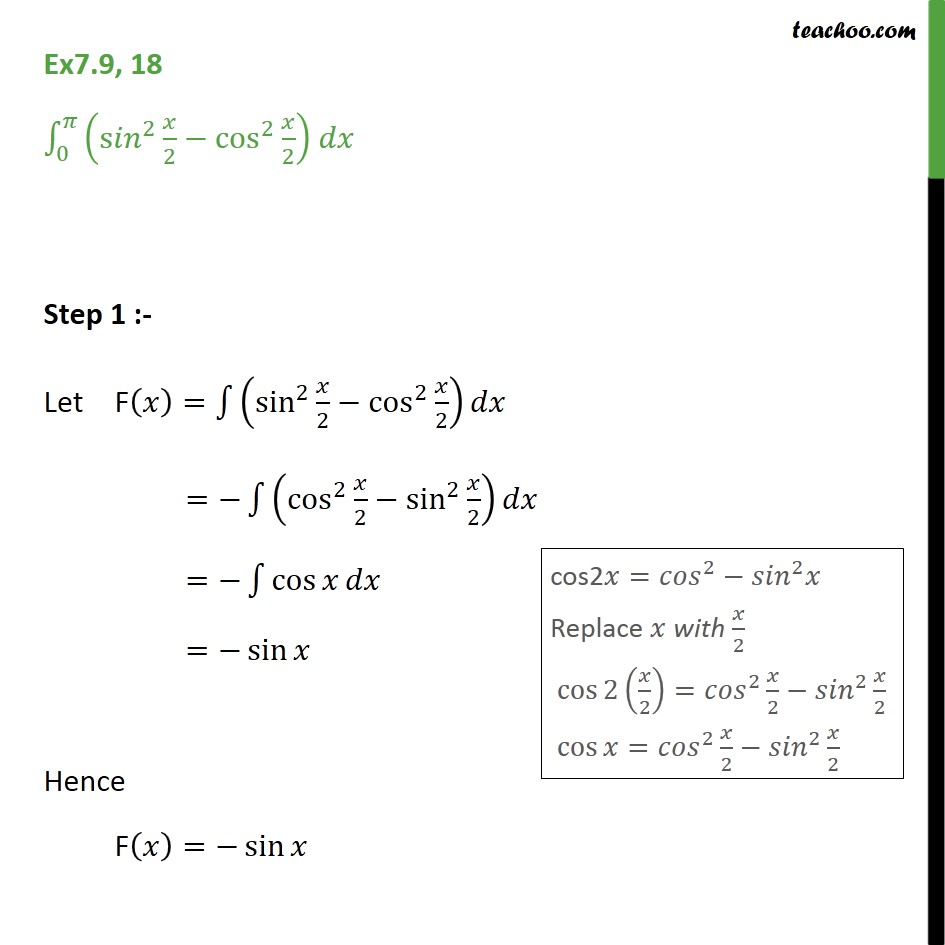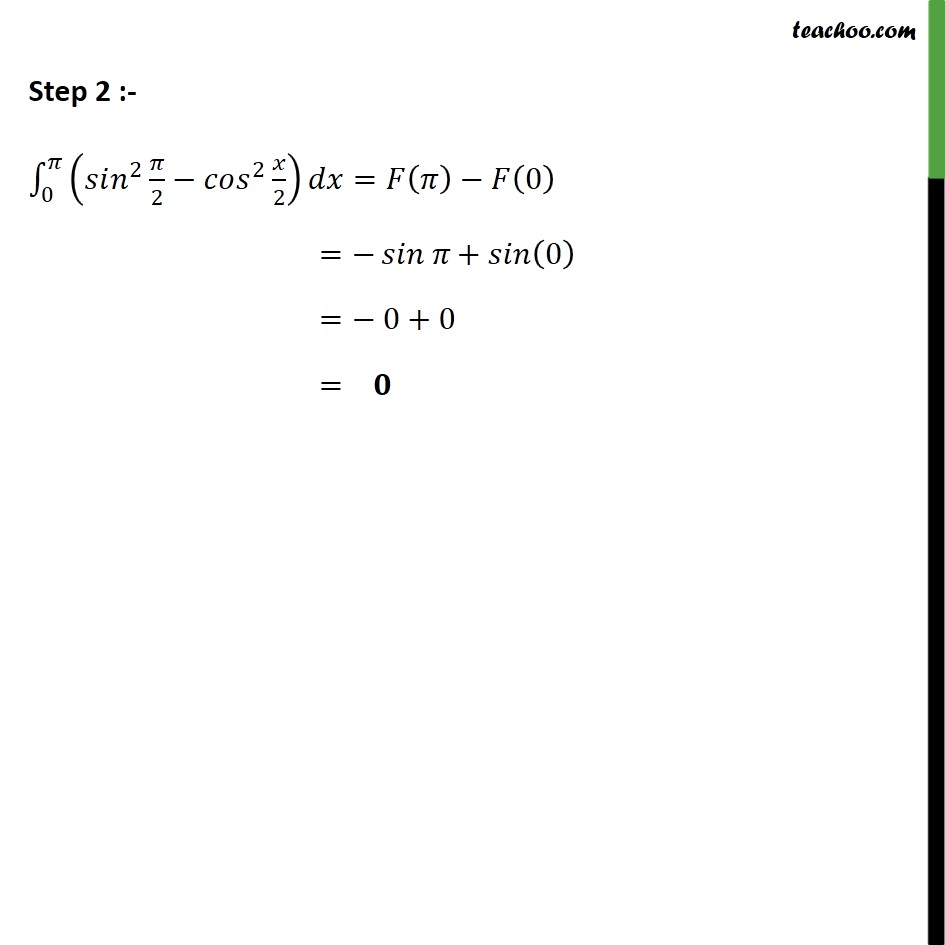Ex 7.9

Chapter 7 Class 12 Integrals
Serial order wiseGet live Maths 1-on-1 Classs - Class 6 to 12

### Transcript

Ex7.9, 18 0 s 2 2 cos 2 2 Step 1 :- Let F = sin 2 2 cos 2 2 = cos 2 2 sin 2 2 = cos = sin Hence F = sin Step 2 :- 0 2 2 2 2 = 0 = + 0 = 0+0 =